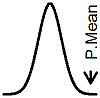P.Mean: Calculating predicted probabilities from a logistic regression model (created 2013-07-31). News: Sign up for "The Monthly Mean," the newsletter that dares to call itself average, www.pmean.com/news.Suppose you run a logistic regression model and want to take the coefficients from that model and do something useful with them. In particular, you want to see what your logistic regression model might predict for the probability of your outcome at various levels of your independent variable. Here's how you would do it.

This example of a logistic regression model is taken from

One of the logistic regression models looks like this.The independent variable is the mother's age in years and the dependent variable is whether the infant was breast feeding at discharge from the hospital. This was a study in pre-term infants, a group where breast feeding is difficult because the mother gets home before the baby does.

The highlights in this table are for a different purpose, and the two numbers that you need to focus on are the slope (0.157) and the intercept (-3.654).

A logistic regression model makes predictions on a log odds scale, and you can convert this to a probability scale with a bit of work. Suppose you wanted to get a predicted probability for breast feeding for a 20 year old mom. The log odds would be

`-3.654+20*0.157 = -0.514`

You need to convert from log odds to odds. Keep in mind that SPSS and most other statistical software will use a natural logartithm (base e) rather than a base 10 logarithm. To move back from the log odds scale to the odds scale you need an antilog, which for the natural logarithm, is an exponential function.

`exp(-0.514) = 0.598`

Now you need to convert from odds to probability.

`0.598 / (1+0.598) = 0.374`

This is a fairly low probability. What would be the predicted probability for a 30 year old mom?

```log odds = -3.654+30*0.157 = 1.06 odds = exp(1.06) = 2.89 prob = 2.89 / (1+2.89) = 0.743```

What is the predicted probability for a 40 year old mom?

```log odds = -3.654+40*0.157 = 2.63 odds = exp(2.63) = 13.9 prob = 13.9 / (1+13.9) = 0.933```

These predicted probabilities have a fair amount of uncertainty associated with them, and you should consider confidence intervals for these predictions. Unfortunately, such intervals are not easy to get in SPSS. If I get a chance, I will try to work out some examples of these intervals.This page was written by Steve Simon and is licensed under the Creative Commons Attribution 3.0 United States License. Need more information? I have a page with general help resources. You can also browse for pages similar to this one at Logistic Regression.